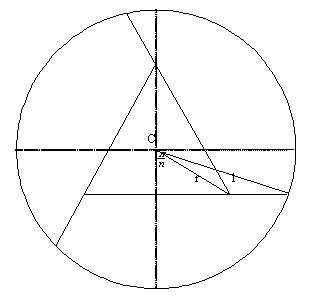#### You may also like### Baby Circle

A small circle fits between two touching circles so that all three circles touch each other and have a common tangent? What is the exact radius of the smallest circle?### Kissing

Two perpendicular lines are tangential to two identical circles that touch. What is the largest circle that can be placed in between the two lines and the two circles and how would you construct it?### Logosquares

Ten squares form regular rings either with adjacent or opposite vertices touching. Calculate the inner and outer radii of the rings that surround the squares.

# Spokes

##### Age 16 to 18Challenge LevelYou know what the area of the four parts must be. Think about symmetrical drawings. Can you draw three lines inside the circle in such a way that they enclose an area which can be expanded or contracted to give the required area? The Solution section gives one possibility (see link above). There are many other possibilities you could investigate. Here is just one alternative line of approach you could pursue: The diagram shows another solution for 3 lines but the same principle applies to any regular polygon. For an $n$-gon the angle marked at the centre of the circle $C$ will be ${\pi \over n}$. As $n$ gets larger the polygons will get smaller and the lines will 'radiate' out more like the spokes of a wheel.

In general, if $L_n$ is the length of the spoke with $n$ spokes, $x_n$ the length of the edge of the polygon in the centre and $r_n$ the radius of the circumcircle of the polygon,then $$L_n = {x_n\over 2} + \sqrt {1 - (r_n\cos {\pi\over n})^2}$$ so that$L_n \to 1$ as $n\to \infty$.\par For $n=3$, $L_n \approx 1.595$; for $n=20$, $L_n\approx 1.011$ and for ${n=30}$, $L_n\approx 1.003$.